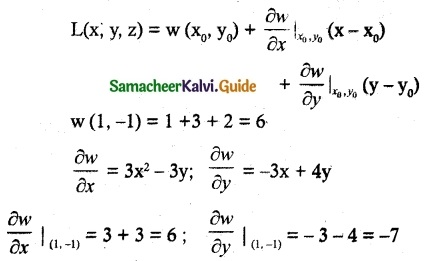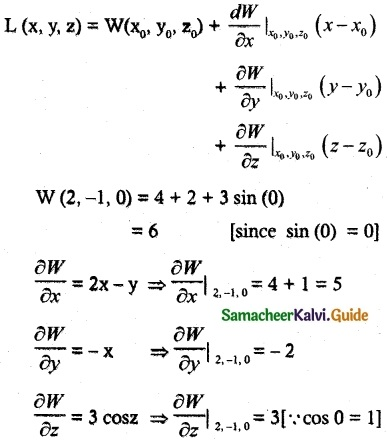Tamilnadu State Board New Syllabus Samacheer Kalvi 12th Maths Guide Pdf Chapter 8 Differentials and Partial Derivatives Ex 8.5 Textbook Questions and Answers, Notes.

## Tamilnadu Samacheer Kalvi 12th Maths Solutions Chapter 8 Differentials and Partial Derivatives Ex 8.5

Question 1.
If w(x, y) = x³ – 3xy + 2y², x, y ∈ R, find the linear approximation for w at (1, -1) linear approximation for w at (1, -1)
Solution:
w(x, y) = x³ – 3xy + 2y², x, y ∈ R, (1, -1)∴ L(x, y, z) = 6 + 6(x – 1) -7(y + 1)
L (x, y, z) = 6x – 7y – 7Question 2.
Let z (x, y) = x² y + 3xy4, x, y ∈ R. Find the linear approximation for z at (2, -1).
Solution:L (x, y, z) = 2 – (x – 2) – 20 (y + 1)
= 2 – x + 2 – 20y – 20
= -x – 20y – 16
= -(x + 20y + 16)Question 3.
If v (x, y) = x² – xy + $$\frac { 1 }{ 4 }$$y² + 7, x, y ∈ R find the differential dv.
Solution:
First let us find vx, vy
Now, vx = $$\frac{\partial v}{\partial x}$$ = 2x – y
vy = $$\frac{\partial v}{\partial y}$$ = -x + $$\frac { 1 }{ 2 }$$ y
The differential is
dv = vx dx + vy dy
dv = (2x – y) dx + ($$\frac { 1 }{ 2 }$$ y – x) dyQuestion 4.
Let W (x, y, z) = x² – xy + 3sinz, x, y, z ∈ R. Find the linear approximation at (2, -1, 0).
Solution:
W (x, y, z) = x² – xy + 3sinz, x, y, z ∈ RL (x, y, z) = 6 + 5 (x – 2)- 2 (y + 1) + 3 (z)
= 6 + 5x – 10 – 2y – 2 + 3z
= 5x – 2y + 3z – 6Question 5.
Let V (x, y, z) = xy + yz + zx, x, y, z ∈ R. Find the differential dV.
Solution:
V(x, y, z) = xy + yz + zx
Vx = y + z
Vy = x + z
Vz = y + x
The differential is dV = (y + z) dx + (x + z) dy + (y + x) dz